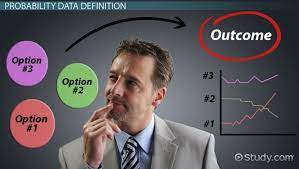After my first and second degrees in computer science, I thought I will not encounter any mathematical subject. Although, I may have to pursue other higher degrees, however, it may not require intense calculations. I thought that MBA is one of those degrees that will have nothing to do with major computations. I have seen some minor computations in some courses like Corporate Financial Accounting. They are mainly additions and subtractions. The introduction of probability in one of our courses – Data Analytics, broke my heart. Some may argue that it is not mathematics, but the definition will explain better.

Definition of Probability

Probability is the branch of mathematics that gives a numerical representation of how likely an event will occur. We apply the concept of probability in our daily activity, especially in decision making, and try to know the possibility of the occurrence of an event by considering possible outcomes. A typical example is the popular ludo game. This is a classical example of probability. When you roll a dice, there is the possibility of getting the numbers 1 to 6, since a dice is a cube with the six sides labelled 1 to 6.

Before we go further, I would like to explain some basic terms in probability.

An experiment is a planned operation carried out under controlled conditions.
Examples of experiments with respect to probability are: Flipping one fair coin twice and throwing dice.

The sample space of an experiment is the set of all possible outcomes.

A result of an experiment is called an outcome. The outcome of flipping a fair coin is a tail or head, while the outcome of throwing dice is 1,2 3,…6

An event is a particular result or set of results amongst the possibilities in the sample space: For example, obtaining 3 from a die or betting a tail in flipping an affair coin is an event.

How is probability assigned?

There are three ways of assigning a probability to an event. They are:

1. The Classical Method
2. Relative Frequency
3. Subjective method.

For now, I will focus more on the classical method.

The classical approach is based on equal likely events. Going by the example of throwing a dice. There are six possible outcomes, and each of these outcomes has an equal likelihood to occur. That means that getting a 1 is equal to the possibility of getting a 2, 3, 4,5 or 6.

Therefore, the formula for the probability of an event equals:

(The number of favourable outcomes) / (the total number of favourable outcomes)

This implies when I throw a dice. The probability of getting a 2 is 1/6.

to explain this, when dice are through, there is only 1 possibility of having the number 2. This is because there is only a single occurrence of 2 in the numbers between 1 and 6.

In this same experiment of throwing a dice, there are 6 possible outcomes which are 1 to 6.

Probability is very important in decision making. Good knowledge of probability enables the manager to analyze the possible options or outcomes in an event, evaluate their risk and make a good prediction

#MMBA 3## Next Generation Fixed Wireless Access (NGFWA) for Service Providers: A Viable Alternative to Traditional Fiber-Optic Networks

in## Transforming Nigeria’s Local Government System for Rural Economic Growth and Increased GDP

in